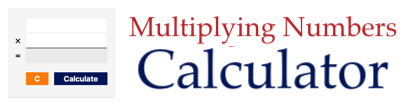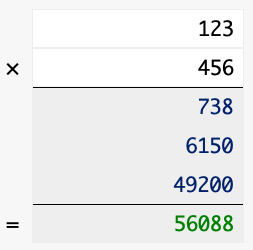Multiplying Numbers CalculatorThis useful Multiplying Numbers Calculator can multiply two numbers together and show you how to do it with the long multiplication method.

Please enter your multiplication problem below for us to solve.

×

We will use an example to explain how our Multiplying Numbers Calculator works. When we enter 123 times 456 into our Multiplying Numbers Calculator, we get the following:1) Multiply 6 by 3 to get 18. Then put 8 below and keep 1 as carryover.

2) Multiply 6 by 2 to get 12 and add carryover to get 13. Then put 3 below and keep 1 as carryover.

3) Multiply 6 by 1 to get 6 and add carryover to get 7. Then put 7 below.

Now we have the first line: 738

4) We are doing the tens place next so add 0 below.

5) Multiply 5 by 3 to get 15. Then put 5 below and keep 1 as carryover.

6) Multiply 5 by 2 to get 10 and add carryover to get 11. Then put 1 below and keep 1 as carryover.

7) Multiply 5 by 1 to get 5 and add carryover to get 6. Then put 6 below.

Now we have the second line: 6150

8) We are doing the hundreds place next so add 00 below.

9) Multiply 4 by 3 to get 12. Then put 2 below and keep 1 as carryover.

10) Multiply 4 by 2 to get 8 and add carryover to get 9. Then put 9 below.

11) Multiply 4 by 1 to get 4. Then put 4 below.

Now we have the third line: 49200

Finally, you simply add up the three lines: 738 + 6150 + 49200 = 56088.

Of course you don't have to do all these steps, because we do it for you! Simply use the Multiplying Numbers Calculator at the top of this page. There you have it folks. Enjoy!

You may also be interested in our Times Tables Test.